## Analytical Calculation of the Scattering Curve I(q) and the Real Space Function p(r) of a Simple Model (Example).

As an example, the scattering curve in reciprocal space and p(r)-function in real space from a model consisting of differently sized spheres are calculated analytically from the Debye-formula in reciprocal space (Debye, P. J. W. (1915), Ann. Phys. 351, 809–823) and its analogue in real-space (Glatter, O. (1980), Acta Phys. Austriaca 52, 243–256). We build a model consisting of 19 spheres (with different radii and constant electron densities) as shown below.The x-y-z coordinates, their respective radii and (optionally different) electron densities of the model are entered as input in the I(q)-p(r)-Debye calculator.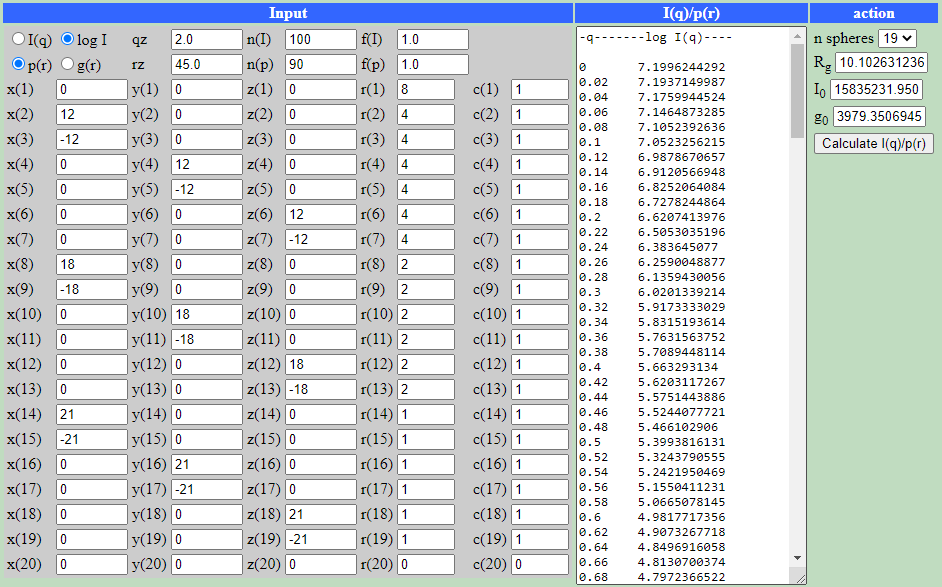The results - I(q) scattering curve and p(r) real-space function of the model (maximum dimension is 44 units) - are shown below: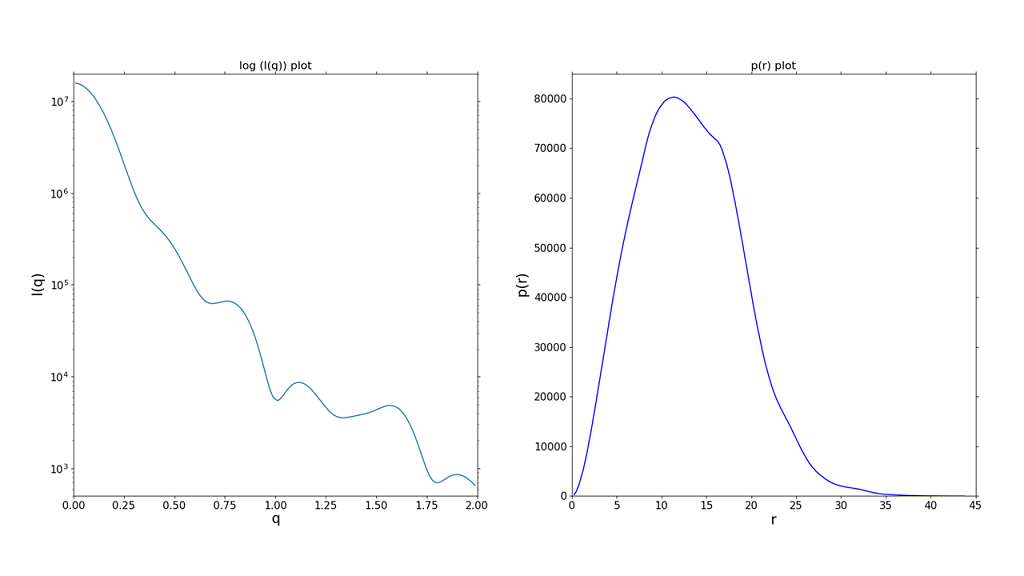An animation of the used model can be seen hereNext, we calculate I(q) and p(r) of 2 different models (each composed of 5 spheres) which have the same histogram of intraparticular distances despite of the different 3D-shape. This results in an identical reciprocal and real space function demonstrating the ambiguity of these one-dimensional functions with respect to the 3D real-space model.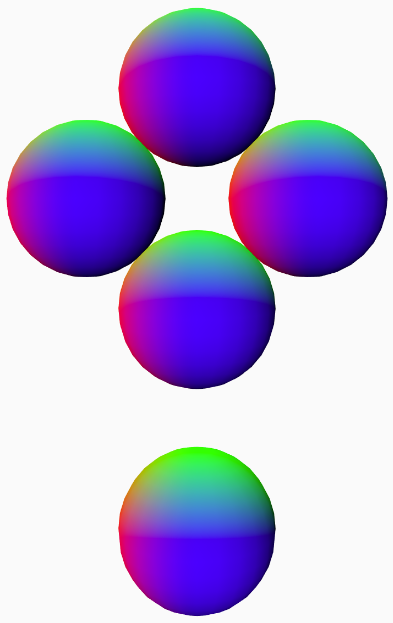Model1 (left) and Model2 (right)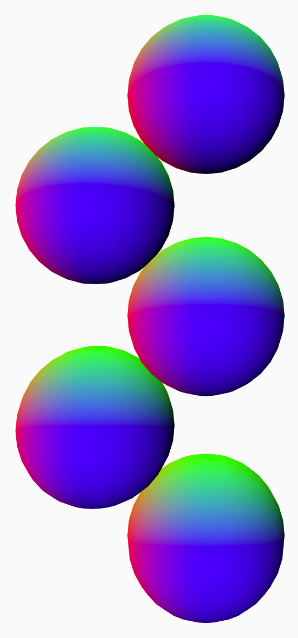Model1 and Model2 are composed of 5 spheres each. The coordinates, radius and electron densities of the two different models are entered in the I(q)-p(r)-Debye-calculator.

Model1: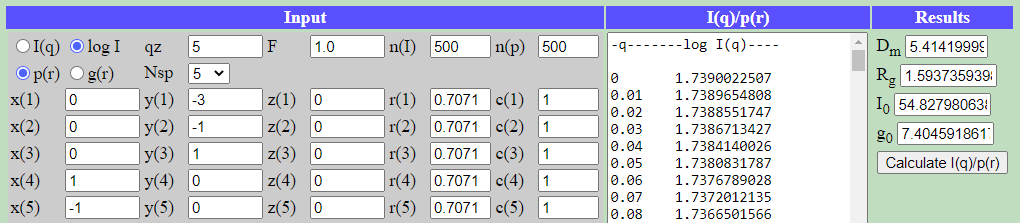Model2: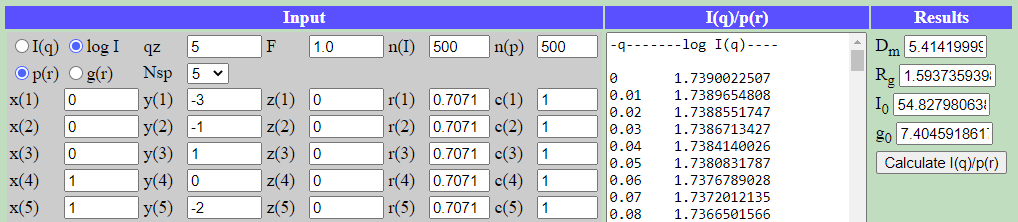Their (identical) scattering curve (left: log(I) vs q) and real space function (right: p(r) vs r) are displayed below: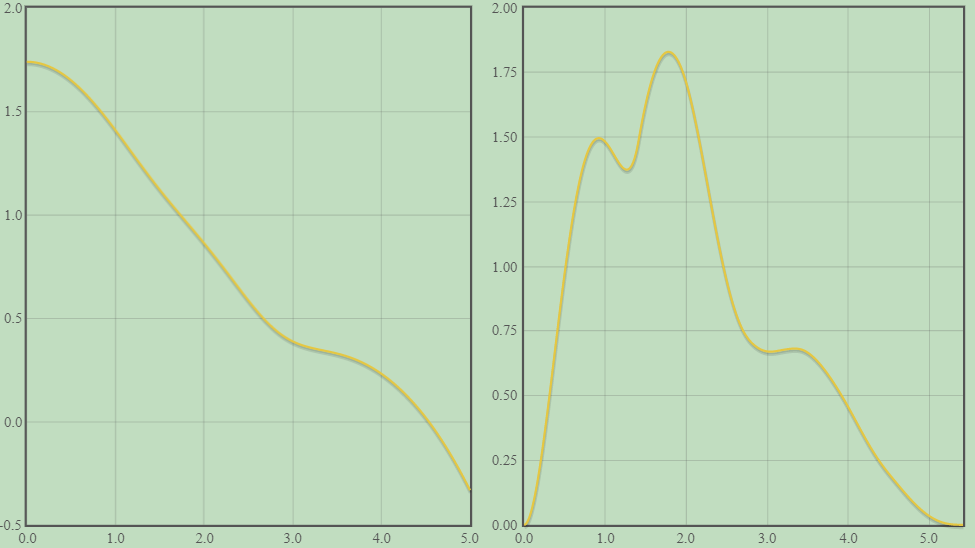Author: M.Kriechbaum, TU-Graz (2020), e-mail: manfred.kriechbaum@tugraz.at